maths > fractions

Proper, Improper, and Mixed fractions

what you'll learn...

overview

In this, the following are explained.

•  proper fraction: smaller than a whole and properly a fraction of a whole

•  improper fraction: larger than a whole in fraction form

•  mixed fraction: larger than a whole given as mix of a whole number and a fraction

mixing proper and improper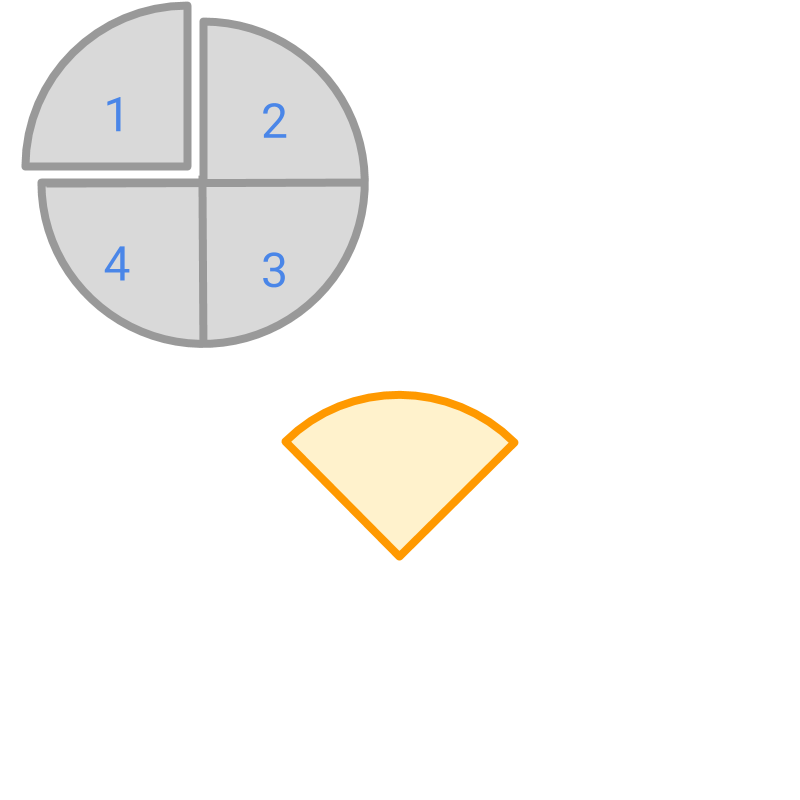Consider the given figure. The colored part is the representation of a fraction and the gray part is shown only as a representation of the whole.

The fraction is $\frac{1}{4}$$\frac{1}{4}$.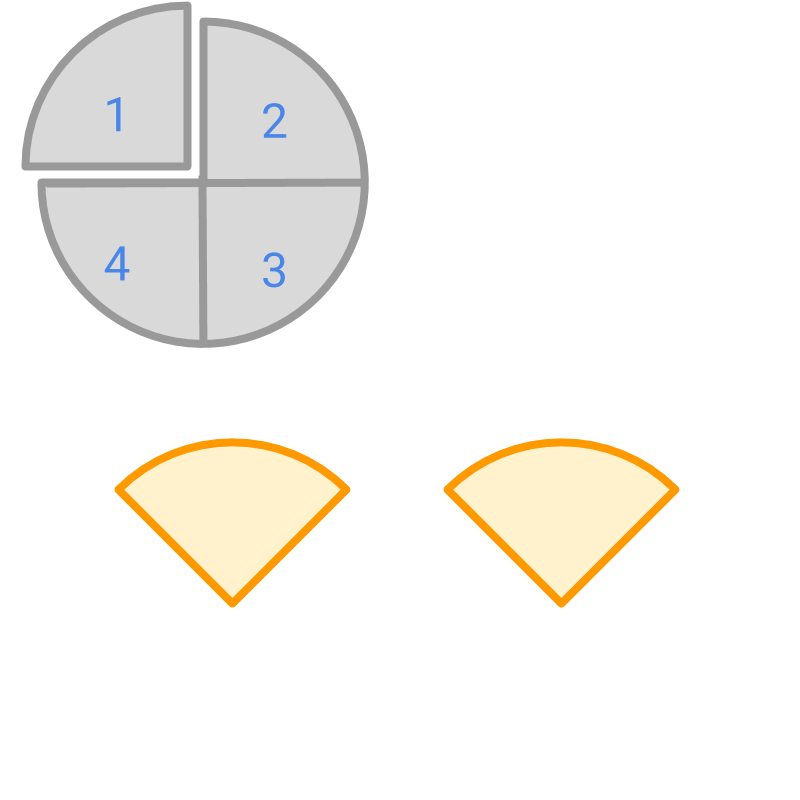The fraction represented by the colored part in the given figure is $\frac{2}{4}$$\frac{2}{4}$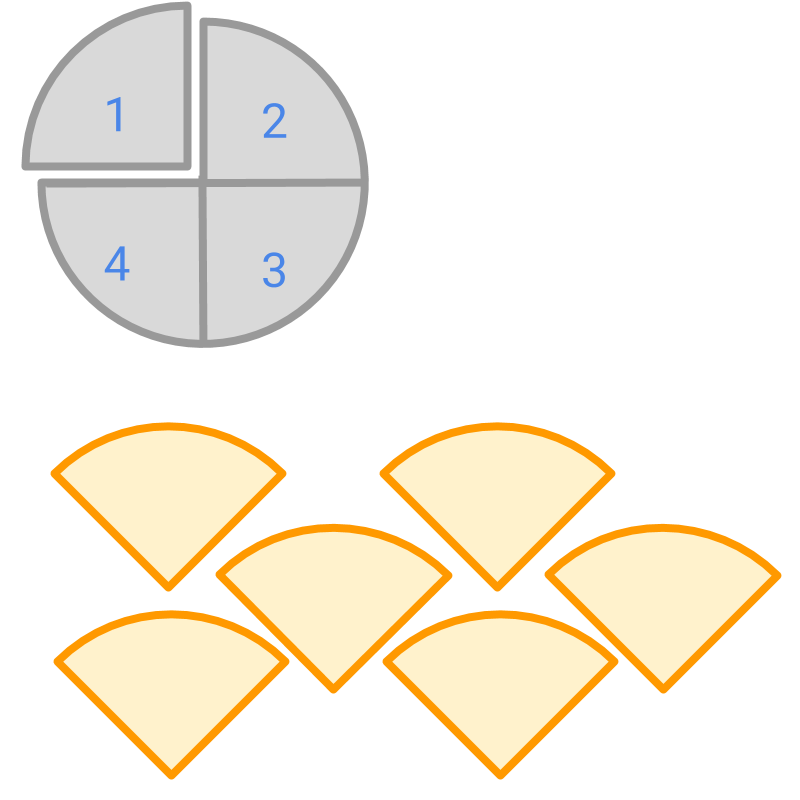The fraction represented by the colored part in the given figure is $\frac{6}{4}$$\frac{6}{4}$

The picture represents the fraction $\frac{6}{4}$$\frac{6}{4}$. Number of parts in the given fraction: $6$$6$
Number of parts making a whole: $4$$4$
The number of parts in the given fraction is greater than the number of parts making a whole.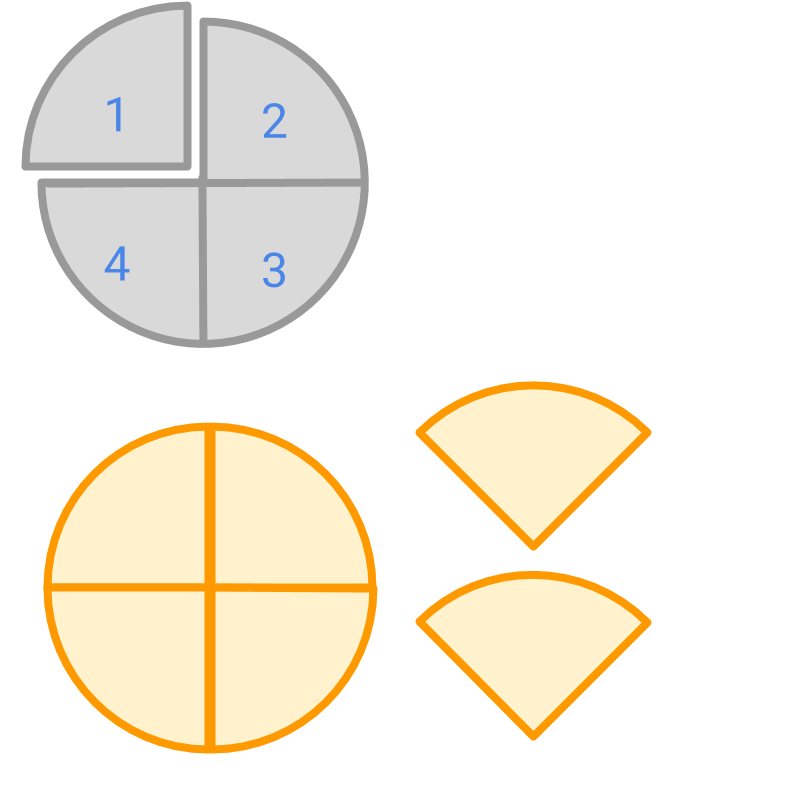The picture represents the fraction $\frac{6}{4}$$\frac{6}{4}$. The $4$$4$ parts of the given fraction is rearranged to make a whole and remaining $2$$2$ parts are shown nearby. So, $\frac{6}{4}$$\frac{6}{4}$ can be given as $1\frac{2}{4}$$1 \frac{2}{4}$.

naming them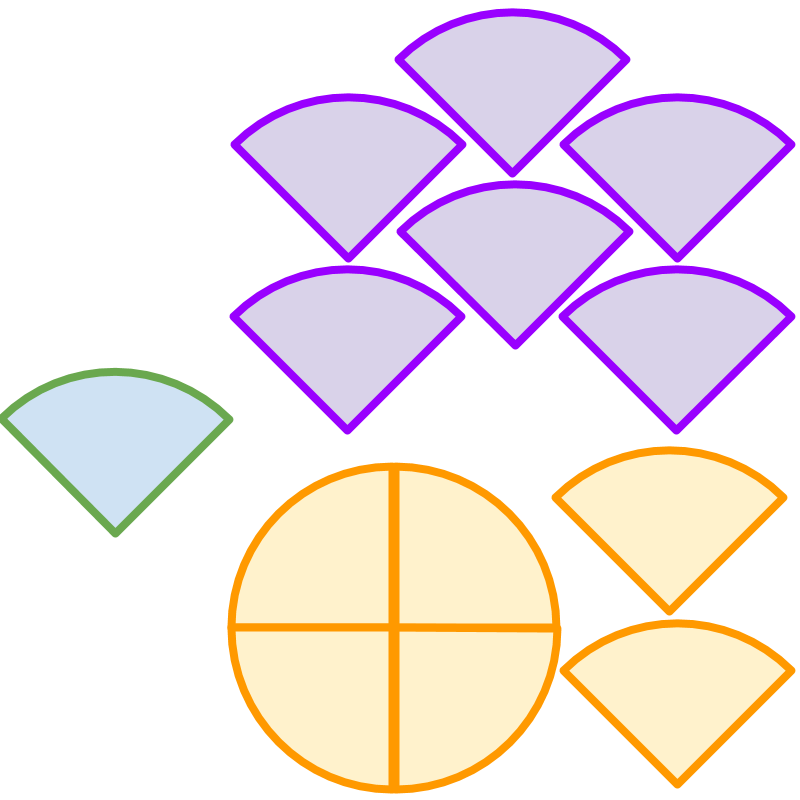The picture represents three fractions

•  Fraction given by green part: $\frac{1}{4}$$\frac{1}{4}$

•  Fraction given by purple parts : $\frac{6}{4}$$\frac{6}{4}$

•  Fraction given by orange parts : $1\frac{2}{4}$$1 \frac{2}{4}$

The word "fraction" means "part of a whole".

•  In the three given fractions, only $\frac{1}{4}$$\frac{1}{4}$ is strictly part of whole. The value of the fraction is less than $1$$1$, which is properly part of whole. Such fractions are called proper fractions.

•  The fraction $\frac{6}{4}$$\frac{6}{4}$ is greater than $1$$1$, and is represented as numerator by denominator. Such fractions are called improper fractions.

•  The fraction $1\frac{2}{4}$$1 \frac{2}{4}$ is greater than $1$$1$, and is represented as a whole number along with a fraction. Such fractions are called mixed fractions.

The word "proper" means: required or correct type. Proper fractions are fractions that are smaller than a whole and properly a fraction of a whole.

The word "improper" means: not of correct type. Improper fractions are fractions that are larger than a whole.

The word "mixed" means: consisting of different quantities or elements. Mixed fractions are fraction that have an integer count of whole and a proper fraction.

Proper Fraction: number of parts in the fraction (numerator) is less than the number of parts making a whole(denominator) given as numerator divided by denominator. Proper fractions are less than 1.

Improper Fraction: number of parts in the fraction (numerator) is greater than the number of parts making a whole(denominator) given as numerator by denominator. Improper fractions are greater than 1 given as numerator by denominator.

Mixed Fraction: Specified as number of wholes with a fraction. Mixed fractions are greater than 1 and given as whole number followed by a numerator by denominator.

examples

What type of fraction is $\frac{3}{7}$$\frac{3}{7}$?

What type of fraction is $\frac{17}{7}$$\frac{17}{7}$?

What type of fraction is $2\frac{3}{7}$$2 \frac{3}{7}$?

What type of fraction is $7\frac{30}{35}$$7 \frac{30}{35}$?

summary

»  Proper Fraction
→  fraction is smaller than the whole
→  eg: $\frac{2}{4}$$\frac{2}{4}$»  Improper Fraction
→  fraction is larger than the whole
→  eg: $\frac{6}{4}$$\frac{6}{4}$»  Mixed Fraction
→  a whole number and a fraction given together
→  eg: $1\frac{2}{4}$$1 \frac{2}{4}$Outline